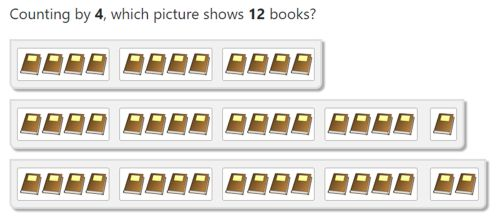# Counting Worksheet Help

#### Inputs

• Description - This optional field is used to identify your online problems. The description is automatically displayed in the header on the page where the student selects problem answers.
• Problem count - This allows you to specify the total number of problems you want the student to complete.
• Count by - This input field indicates what number(s) you want the student to count by. More than a single number can be counted. For example, if you want to count by only 2's, enter a "2". If you want problems that count by 2 and 3, you can enter "2,3". You are not allowed to count by 0.

If you receive an "Invalid Count by, Count from, Count to" error, this is the result of the Count from and Count to being too close, or the largest Count by value being too large. Try adjusting these input values to eliminate the error (i.e. increase the Count to value).

• Count from - This is the starting value you want in the student problems. For example, if you input "5", then the student will be presented with problems where the answer is between 5 and the Count to.
• Count to - This is the largest value you want in the student problems. For example, if you input "20", then the student will be presented with problems where the answer is between your Count from number and 20.
• Pictures - Select a category from the picture list to indicate the type of pictures that are displayed in each problem. A randomly selected picture from the category is automatically choosen for each problem.

#### Options

• Confirm answers - If this option is selected, before moving on to the next problem, the student will be provided immediate feedback on whether their selected answer was correct.
• Allow partial counts - By selecting this feature, the student will be presented problems that may not be an exact multiple of the "Count By" numbers. For example, if you are counting by 4's then the student may be asked to solve a problem where the answer is 12. Here is an example of counting by 4's, but the second and third choices are 17 and 18 (i.e. not multiples of 4):#### Re-Shuffle

For each set of options you select on the build page, you will get the same problems each time you click the Generate! button. If you are not satisfied with the problems generated for you, then you can click the Re-Shuffle button. Once you click Re-Shuffle, you can re-click the Generate! button to generate a new set of problems. There is no limit to the number of times you can re-shuffle your problems.